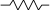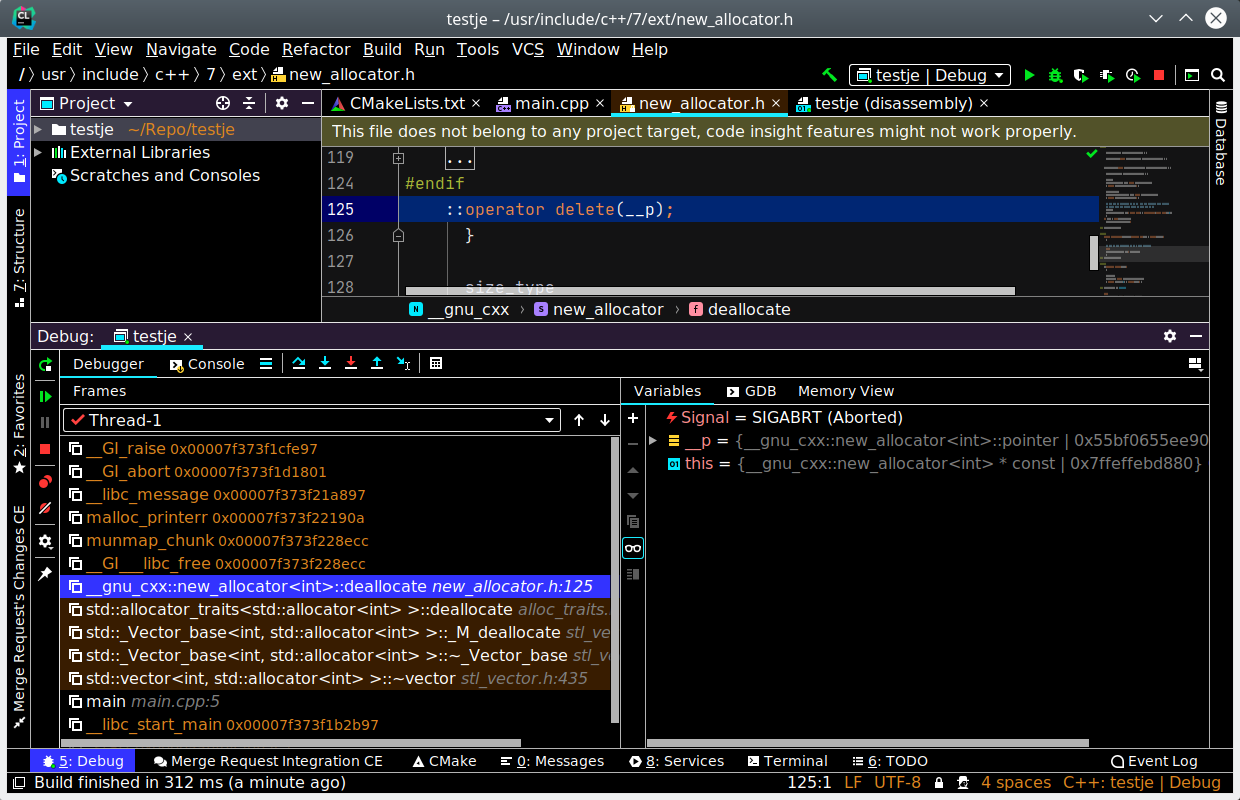# Raymii.orgQuis custodiet ipsos custodes?
Home | About | All pages | Cluster Status | RSS Feed | Gopher

## Here be dragons, or, invalidated iterators

Published: 03-05-2020 | Author: Remy van Elst | Text only version of this article

❗ This post is over two years old. It may no longer be up to date. Opinions may have changed.

Recently I had a new "first-time" moment. You know the ones, the, "oh right", moments, after you put in a bit of research. Mine was, as you might expect from all the other recent content, related to C++. I learned, the hard way, that ```iterator-based for loops``` don't like to be resized during the loop. Well, they don't really care, but some precautions are to be taken since the `iterator` used in the loop might be invalidated. Or as the very helpfull error during the crash prints to the console, `munmap_chunk(): invalid pointer` and your debugger points you to somewhere deep in `new_allocator.h`. In this article I'll give a few examples, both using index based for loops and iterator based for loops, plus some more details on what's going on with iterator invalidation.

Here's a picture of the screen that CLion, my editor of choice gave when the crash occurs:The crash only occured when I used an iterator based for loop, not when I used a index based for loop, leaving the rest of the code unchanged. As I'd never seen this happen before and never seen or heard of iterator invalidation before, it was quite the learning experience. Lots of information available on interator invalidation, this page on cppreference has an overview of what operations invalidate an iterator for what type of container you use.

### Iterators

Back to the beginning, a brief overview of iterators. The best simple description I could find is the following one:

Iterator: a pointer-like object that can be incremented with `++`, dereferenced with `*`, and compared against another iterator with `!=`.

Every STL container provides iterators, and if you make your own containers, it's beneficial to also make sure that, if applicable, also can be iterated over. This allows you to make more generic code, or later on change the underlying implementation without also changing all the users of the code (assuming they use iterators).

For example, the following index based `for` loop works for a `std::vector`:

``````std::vector<int> v {0, 1, 2, 3, 4, 5};
for (size_t i = 0; i < v.size(); ++i) {
std::cout << v.at(i) << " ";
}
``````

Output:

``````0 1 2 3 4 5
``````

This form of looping only works on sequential random access containers like `std::vector` or `std::array`, but not for a `std::list`, or an associative container like `std::map`.

The equivalent iterator based for loop looks like this:

``````std::vector<int> v {0, 1, 2, 3, 4, 5};
for (auto it = v.begin(); it != v.end(); ++it) {
std::cout << *it << " ";
}
``````

Output:

``````0 1 2 3 4 5
``````

You access the current element via the `*` dereference operator, like a pointer. Also note that the conditional expression in the for loop (`it != v.end()`) is an equality comparison whereas the indexed for loop uses an less-than comparison. The reason why is explained here quite well.

The above format can also be expressed in a range based for loop:

``````std::vector<int> v {0, 1, 2, 3, 4, 5};
for (int & i : v) {
std::cout << i << " ";
}
``````

To summarize, if you are iterating with an index you are assuming:

• that its content is ordered
• that its content can be obtained by an index
• that the index increment will hit every item
• that the index starts at zero

With an iterator, you are saying `give me everything so I can work with it`.

### Iterator invalidation and for loops

If you understand how pointers work and why you shouldn't write to pointers that have been deleted, you can skip this section. Otherwise, if you, like me, had a bit of trouble with grasping iterator invalidation, read on.

A for loop, as described here, often has three parts:

``````for ( init statement; condition ; iteraton expression)
statement
``````

The first part is often the assignment (`size_t i = 0`, `auto it = v.begin();`). The second part is the check if the loop has to stop (`i < v.size()`, `it != v.end()`) and the third part is what the loop has to do if the check is not true yet (`++i`, `++it`).

The `init statement` is executed only once. The `condition` and `iteration expression` are executed repeatedly (before each iteration) until the value of `condition` becomes `false`.

Just for fun, think about what would happen if the init statement was also executed before every iteration. How could a loop ever work if that happened.

• The iterator `auto it = v.begin()` is sort of a glorified pointer.
• If you do something to the vector inside the loop, `it` might point to memory that no longer contains the vector.
• Resizing a vector, or doing a `push_back` inside the loop, might result in:
• A new, bigger vector being allocated
• The elements copied from the old vector to the new vector
• The old vector being deleted.
• The `it` iterator (that was assigned in the init statement in the for loop), is still pointing to the memory containing the old vector.
• It is unaware a new bigger vector in a different location is now being used,
• Unless you explicitly tell it by updating the iteraror.

### Example code

The code I wrote had to do something with every element in the vector, and if the last element matched a set of conditions, it should add one more element to the vector. The index based for loop example:

``````std::vector<int> v {0, 1, 2, 3, 4, 5};
for (size_t i = 0; i < v.size(); ++i) {
if (v.at(i) == 5 and (i+1) == v.size()) {
v.resize(v.size() + 1);
v.at(i + 1) = 999;
v.at(i) = 0;
}
}
``````

If the last element is `5`, then add a new element `999` and set the current element to `0`.

The iterator based example, that crashes:

``````std::vector<int> v {0, 1, 2, 3, 4, 5};
for (auto it = v.begin(); it != v.end(); ++it) {
if (*it == 5 && std::next(it) == v.end()) {
v.resize(v.size() + 1);
*std::next(it) = 999;
*it = 0;
}
}
``````

The fix is quite simple, we must explicitly tell the iterator that it has changed. In my case I set the iterator to the current element (`v.size() - 2`). The next loop iteration then continues with the new element.

``````std::vector<int> v {0, 1, 2, 3, 4, 5};
for (auto it = v.begin(); it != v.end(); ++it) {
if (*it == 5 && std::next(it) == v.end()) {
v.resize(v.size() + 1);
it = std::next(v.begin(), v.size() - 2);
*std::next(it) = 999;
*it = 0;
}
}
``````

### Conclusion

Now that I grasp it all, the entire concept is simple and clear. But, isn't that always the case when you've lost something, it is is always in the last location you look for it. Unfortunately peanut butter.

Tags: blog , c++ , cpp , development , for , iterator , loop , pointer